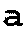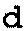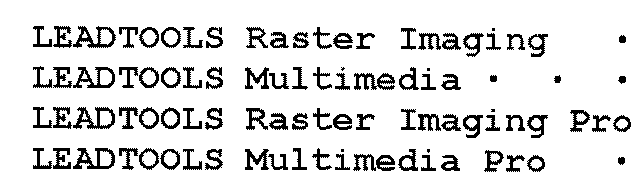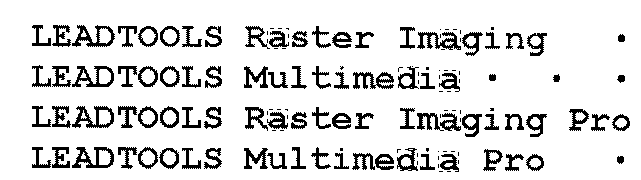﻿ CorrelationListCommand Class - Namespace: Leadtools.ImageProcessing.Core - Assembly: Leadtools.ImageProcessing.Core - Raster, Medical, Document Help
←Select platform
In This Topic ▼

# CorrelationListCommand Class

Summary

Compares the images in a list with all the areas of the same dimensions in an image and finds those portions that match according to the measure of correlation. This method is only available in the Document/Medical toolkits.

Syntax
C#
VB
Java
Objective-C
C++
``public class CorrelationListCommand : RasterCommand ``
````Public Class CorrelationListCommand  `
`   Inherits RasterCommand ````
``@interface LTCorrelationListCommand : LTRasterCommand ``
``public class CorrelationListCommand extends RasterCommand ``
``public ref class CorrelationListCommand : public RasterCommand   ``

Remarks
• This method compares the images in a list with all the areas of the same dimensions in image and finds those areas that match according to the measure of correlation.
• All the list images must have the same dimensions. The dimensions must be less than or equal to the image dimensions.
• Correlation is a measure of association (resemblance) between two images. It varies from 0 (zero resemblance) to 100 (perfect resemblance).
• This method updates the Points parameter with the point of origin for those areas of the image where the resemblance with an image in the list is greater than the value of Threshold. It also updates ListIndex with the index of that image.
• For example:

1. Select images of the objects for which you want to look and put them in a list For example:2. Select an image that contains the objects for which you are searching. For example:3. Set XStep = 1, YStep = 1 and Threshold = 90 then run the command

4. Points array will be updated with the point of origin (top-left) for each area of the image where the correlation with an image in the list is greater than the correlation Threshold. The height and width of the rectangles are the same as for list images.
5. The result• This method supports 12 and 16-bit grayscale and 48 and 64-bit color images. Support for 12 and 16-bit grayscale and 48 and 64-bit color images is available only in the Document/Medical toolkits.

• If the image has a region, this method works only on the region. If the image does not have a region, this method works on the entire image.
• This method does not support signed data images.
• This command does not support 32-bit grayscale images.

Example

Run the CorrelationListCommand on an image.

C#
VB
````using Leadtools; `
`using Leadtools.Codecs; `
`using Leadtools.ImageProcessing; `
`using Leadtools.ImageProcessing.Core; `
` `
`public void CorrelationListCommandExample() `
`{ `
`   // Load an image `
`   RasterCodecs codecs = new RasterCodecs(); `
`   codecs.ThrowExceptionsOnInvalidImages = true; `
` `
`   RasterImage image = codecs.Load(Path.Combine(LEAD_VARS.ImagesDir, "clean.tif")); `
` `
`   CorrelationListCommand command = new CorrelationListCommand(); `
`   CopyRectangleCommand copyRectangle = new CopyRectangleCommand(); `
` `
`   LeadRect rc_cor = new LeadRect(458, 591, 21, 28); `
`   copyRectangle.Rectangle = rc_cor; `
`   copyRectangle.Run(image); `
`   command.CorrelationImage = copyRectangle.DestinationImage.Clone(); `
` `
`   rc_cor = new LeadRect(507, 591, 21, 28); `
`   copyRectangle.Rectangle = rc_cor; `
`   copyRectangle.Run(image); `
`   command.CorrelationImage.AddPage(copyRectangle.DestinationImage.Clone()); `
` `
`   command.Points = new LeadPoint; `
`   command.ListIndex = new int; `
`   command.Threshold = 90; `
`   command.XStep = 1; `
`   command.YStep = 1; `
`   command.Run(image); `
` `
`} `
` `
`static class LEAD_VARS `
`{ `
`   public const string ImagesDir = @"C:\Users\Public\Documents\LEADTOOLS Images"; `
`} ````
````Imports Leadtools `
`Imports Leadtools.Codecs `
`Imports Leadtools.ImageProcessing `
`Imports Leadtools.ImageProcessing.Core `
` `
`Public Sub CorrelationListCommandExample() `
`   ' Load an image `
`   Dim codecs As RasterCodecs = New RasterCodecs() `
`   codecs.ThrowExceptionsOnInvalidImages = True `
` `
`   Dim image As RasterImage = codecs.Load(Path.Combine(LEAD_VARS.ImagesDir, "clean.tif")) `
` `
`   Dim command As CorrelationListCommand = New CorrelationListCommand() `
`   Dim copyRectangle As CopyRectangleCommand = New CopyRectangleCommand() `
` `
`   Dim rc_cor As LeadRect = New LeadRect(458, 591, 21, 28) `
`   copyRectangle.Rectangle = rc_cor `
`   copyRectangle.Run(image) `
`   command.CorrelationImage = copyRectangle.DestinationImage.Clone() `
` `
`   rc_cor = New LeadRect(507, 591, 21, 28) `
`   copyRectangle.Rectangle = rc_cor `
`   copyRectangle.Run(image) `
`   command.CorrelationImage.AddPage(copyRectangle.DestinationImage.Clone()) `
` `
`   Dim points(30) As LeadPoint `
`   Dim listIndex(30) As Integer `
` `
`   command.Points = points `
`   command.ListIndex = listIndex `
`   command.Threshold = 90 `
`   command.XStep = 1 `
`   command.YStep = 1 `
`   command.Run(image) `
` `
`End Sub `
` `
`Public NotInheritable Class LEAD_VARS `
`   Public Const ImagesDir As String = "C:\Users\Public\Documents\LEADTOOLS Images" `
`End Class ````
````c#[Silverlight C# Example] `
`using Leadtools; `
`using Leadtools.ImageProcessing; `
`using Leadtools.Examples; `
`using Leadtools.Codecs; `
`using Leadtools.ImageProcessing.Core; `
` `
`public void CorrelationListCommandExample(RasterImage image, Stream outStream) `
`{ `
`   CorrelationListCommand command = new CorrelationListCommand(); `
`   CopyRectangleCommand copyRectangle = new CopyRectangleCommand(); `
` `
`   LeadRect rc_cor = new LeadRect(327, 378, 22, 28); `
`   copyRectangle.Rectangle = rc_cor; `
`   copyRectangle.Run(image); `
`   command.CorrelationImage = copyRectangle.DestinationImage.Clone(); `
` `
`   rc_cor = new LeadRect(283, 378, 22, 28); `
`   copyRectangle.Rectangle = rc_cor; `
`   copyRectangle.Run(image); `
`   command.CorrelationImage.AddPage(copyRectangle.DestinationImage.Clone()); `
` `
`   command.Points = new LeadPoint; `
`   command.ListIndex = new int; `
`   command.Threshold = 90; `
`   command.XStep = 1; `
`   command.YStep = 1; `
`   command.Run(image); `
` `
`   // Save result image `
`   RasterCodecs codecs = new RasterCodecs(); `
`   codecs.Save(image, outStream, RasterImageFormat.Jpeg, 24); `
`   image.Dispose(); `
`} `
`vb[Silverlight VB Example] `
`Imports Leadtools `
`Imports Leadtools.ImageProcessing `
`Imports Leadtools.Codecs `
`Imports Leadtools.ImageProcessing.Core `
` `
`Public Sub CorrelationListCommandExample(ByVal image As RasterImage, ByVal outStream As Stream) `
`   Dim command As CorrelationListCommand = New CorrelationListCommand() `
`   Dim copyRectangle As CopyRectangleCommand = New CopyRectangleCommand() `
` `
`   Dim rc_cor As LeadRect = New LeadRect(327, 378, 22, 28) `
`   copyRectangle.Rectangle = rc_cor `
`   copyRectangle.Run(image) `
`   command.CorrelationImage = copyRectangle.DestinationImage.Clone() `
` `
`   rc_cor = New LeadRect(283, 378, 22, 28) `
`   copyRectangle.Rectangle = rc_cor `
`   copyRectangle.Run(image) `
`   command.CorrelationImage.AddPage(copyRectangle.DestinationImage.Clone()) `
` `
`   command.Points = New LeadPoint(29) {} `
`   command.ListIndex = New Integer(29) {} `
`   command.Threshold = 90 `
`   command.XStep = 1 `
`   command.YStep = 1 `
`   command.Run(image) `
` `
`   ' Save result image `
`   Dim codecs As RasterCodecs = New RasterCodecs() `
`   codecs.Save(image, outStream, RasterImageFormat.Jpeg, 24) `
`   image.Dispose() `
`End Sub ````

Requirements

Help Version 20.0.2019.3.12
Products | Support | Contact Us | Intellectual Property Notices